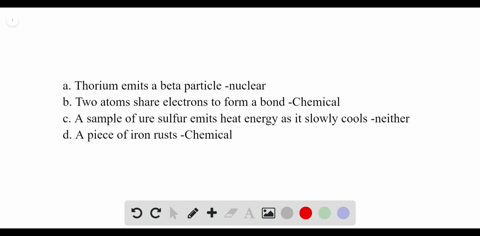### Classify each of the following as a chemical reac…

00:46University of Miami
Problem 26

State what quantities are conserved when balancing a nuclear reaction.

Mass number and atomic number.

## Discussion

You must be signed in to discuss.

## Video Transcript

and I will work on problem 26 from Chapter four in this problem, were asked what is to identify what's conserved in when we're balancing a nuclear reaction. So when you're balancing a nuclear reaction, normally when we balance it regular chemical reaction, we want to balance the number of each Adams on each side. But in a nuclear reaction, the identity of the atom is changing from one element to another. So we don't want to conserve the number of elements on both sides, because the lamentable change, but in a nuclear reaction, we want to conserve mass number because we're gonna have the same number of protons and neutrons on both sides and also atomic number, because we're gonna have the same number of protons on both sides of the reaction. So these two, um, values must be conserved in a nuclear reactor